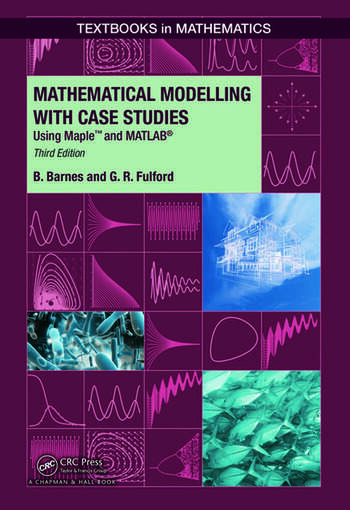# Mathematical Modelling with Case Studies: Using Maple and MATLAB, Third Edition

## 3rd Edition

B. Barnes, G..R. Fulford

Chapman and Hall/CRC
Published December 16, 2014
Textbook - 388 Pages - 143 B/W Illustrations
ISBN 9781482247725 - CAT# K23646
Series: Textbooks in Mathematics

For Instructors Request Inspection Copy

USD\$110.00

FREE Standard Shipping!

## Preview

### Summary

Mathematical Modelling with Case Studies: Using Maple™ and MATLAB®, Third Edition provides students with hands-on modelling skills for a wide variety of problems involving differential equations that describe rates of change. While the book focuses on growth and decay processes, interacting populations, and heating/cooling problems, the mathematical techniques presented can be applied to many other areas.

The text carefully details the process of constructing a model, including the conversion of a seemingly complex problem into a much simpler one. It uses flow diagrams and word equations to aid in the model-building process and to develop the mathematical equations. Employing theoretical, graphical, and computational tools, the authors analyze the behavior of the models under changing conditions. The authors often examine a model numerically before solving it analytically. They also discuss the validation of the models and suggest extensions to the models with an emphasis on recognizing the strengths and limitations of each model.

The highly recommended second edition was praised for its lucid writing style and numerous real-world examples. With updated Maple™ and MATLAB® code as well as new case studies and exercises, this third edition continues to give students a clear, practical understanding of the development and interpretation of mathematical models.

#### Instructors

We provide complimentary e-inspection copies of primary textbooks to instructors considering our books for course adoption.